Bicorn
BICORN

Balmoral Software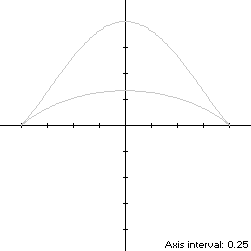The bicorn is a y-symmetric closed curve S with a shape reminiscent of the bicorne hat worn by Napoleon and others. It is defined by the parametric equations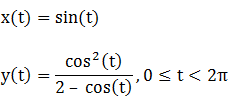The curve follows a clockwise path as t increases, starting from its maximum ordinate at (x(0),y(0)) = (0,1), reaching its maximum abscissa (1,0) at t = π/2, then the local height maximum (0,1/3) at t = π and the minimum abscissa (-1,0) at t = 3π/2 before returning to the y-axis. Since two ordinate minima occur on the x-axis, the bicorn is non-convex by the multiple local extrema test, and the width x height of its bounding rectangle is 2 x 1.

### Metrics

The perimeter of the bicorn is approximately 5.056530 and its area is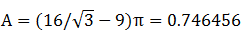Its centroid ordinate is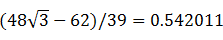The bounding rectangle for inconics is delimited by the lower y-intercept y(t) = 1/3 occurring when t = ±Arccos(2/3) and the corresponding outer abscissas### Convex Hull

The convex hull is created simply by connecting the two cusps of the bicorn with a horizontal line segment of length 2. We have
x'(t) = cos(t)

y'(t) = sin(t){1 - 4/[cos(t) - 2]2},

so by (L1), the perimeter of the convex hull is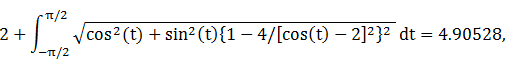which is about 3% shorter than that of the bicorn.

By (A1), the area A of the convex hull is determined by integrating over the same subinterval of T: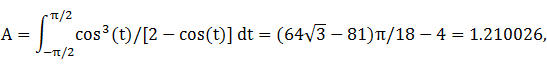which is about 62% larger than the area of the bicorn.

By (C1), the centroid ordinate of the convex hull is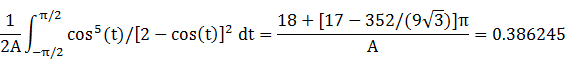### Incircle

A candidate incircle is located between the ordinate extrema of S on the y-axis, and so has radius R = 1/3 and center ordinate d = 2/3. For verification, we have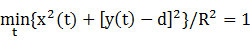### Inellipse

Using z = 1/3 in Lemma E,
d/dt x(t)[y(t) - z] = d/dt sin(t){cos2(t)/[2 - cos(t)] - 1/3}
has a zero at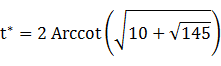The corresponding coordinates are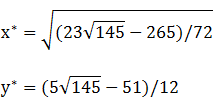We then have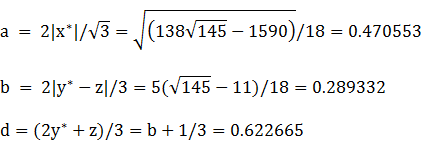For verification,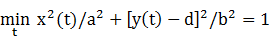### Circumellipse

Using z = 1 in Lemma E,
d/dt x(t)[y(t) - z] = d/dt sin(t){cos2(t)/[2 - cos(t)] - 1}
has a zero at t* = π/2. The corresponding coordinates are
x* = 1

y* = 0,

from which we have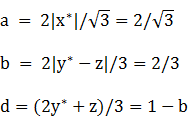For verification,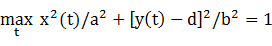### Circumcircle

Since the abscissa extrema are on the x-axis and the bounding rectangle is wider than it is tall, a candidate for the circumcircle has radius 1 and center at the origin. For verification, we have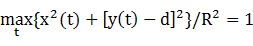### Summary Table

 Perimeter Area Centroid Figure Parameters Incircle R = 1/3 2.094395 0.349066 (0,0.666667) Inellipse a = 0.470553b = 0.289332 2.421315 0.427715 (0,0.622665) Bicorn Width: 2Height: 1 5.056530 0.746456 (0,0.542011) Convex hull 4.90528 1.210026 (0,0.386245) Circumellipse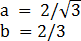5.825171 2.418399 (0,0.333333) Circumcircle R = 1 6.283185 3.141593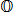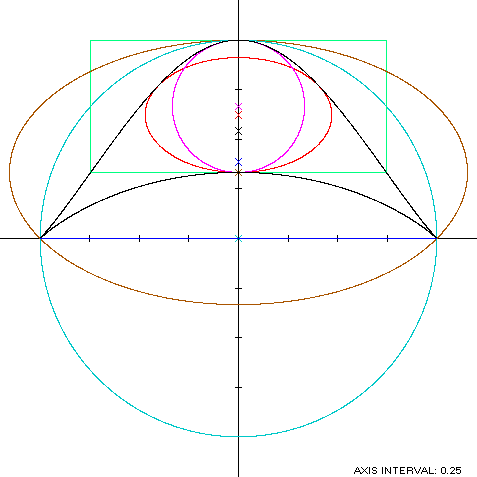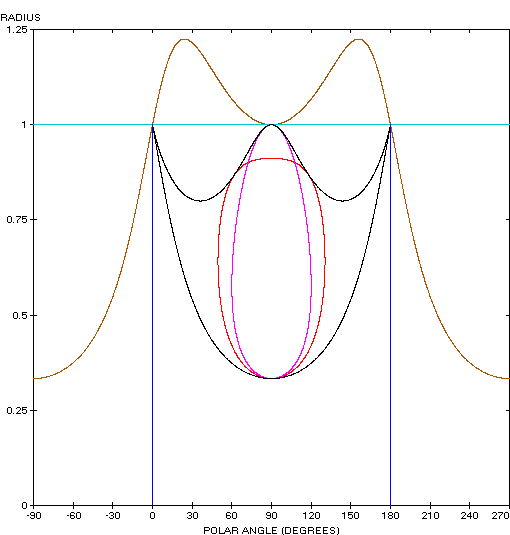Koala Frog